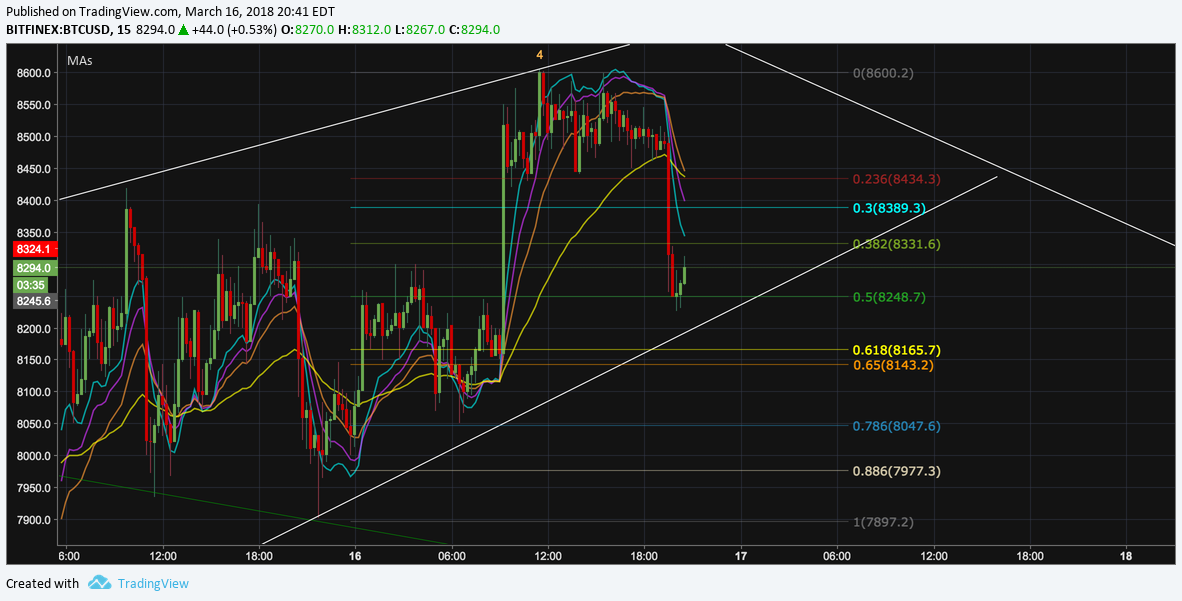Hey Everyone :)

I know how TradingView can be a pain in the ass in regards to adding indicators. If you want to add more than 3 indicators on the free version (like the picture above), you need a loophole around it!

Luckily I have some code (not my code, but I can't find the original author - if it's you, let me know and I'll tag you in the post) that you can easily paste into the Pine Editor section that'll give you access to all your moving averages.

Here it is:

``````
study(title="Moving Averages", shorttitle="MAs", overlay=true)
exponential = input(true, title="Exponential MA")

src = close

ma8 = exponential ? ema(src, 8) : sma(src, 8)
ma13 = exponential ? ema(src, 13) : sma(src, 13)
ma21 = exponential ? ema(src, 21) : sma(src, 21)
ma55 = exponential ? ema(src, 55) : sma(src, 55)

plot( ma8, color= #00e6e6, style=line, title="MMA8", linewidth=3)
plot( ma13, color= #d633ff, style=line, title="MMA13", linewidth=3)
plot( ma21, color=#ff9933, style=line, title="MMA21", linewidth=3)
plot( ma55, color= yellow, style=line, title="MMA55", linewidth=3)
``````

If you want to replace the 8, 13, 21, or 55 EMA all you have to do is...

## 1. Find this section in the code

``````ma8 = exponential ? ema(src, 8) : sma(src, 8)
ma13 = exponential ? ema(src, 13) : sma(src, 13)
ma21 = exponential ? ema(src, 21) : sma(src, 21)
ma55 = exponential ? ema(src, 55) : sma(src, 55)
``````

## 2. Replace the numbers beside "src" to EMA you want to add

``````ma8 = exponential ? ema(src, 100) : sma(src, 100)
``````

Just like that, you have access to all of the EMAs you need in one handy little indicator. Hopefully, this helped you out a bit :) If it did, please resteem and upvote & show your dude some love!